Courses

# Ex 1.4 NCERT Solutions - Real Numbers Class 10 Notes | EduRev

## Class 10 : Ex 1.4 NCERT Solutions - Real Numbers Class 10 Notes | EduRev

The document Ex 1.4 NCERT Solutions - Real Numbers Class 10 Notes | EduRev is a part of the Class 10 Course Class 10 Mathematics by VP Classes.
All you need of Class 10 at this link: Class 10

Exercise 1.4
Ques 1: Without actually performing the long division, state whether the following rational numbers will have a terminating decimal expansion or a non-terminating repeating decimal expansion:
(i) 13/ 3125

(ii) 17/18
(iii) 64/455
(iv) 15/1600
(v) 29/343
(vi) 23/2353

(vii) 129/22577
(viii) 16/15
(ix) 35/50
(x)  77/ 210
Sol:
(i) 13/3125
∵  3125 =  5 × 5 × 5 × 5 × 5 = 55
=  20 × 55,    |∵ 20 = 1
which is of the form (2n · 5m)
∴ 13/3125 is a terminating decimal.
i.e., 13/3125 will have a terminating decimal expansion.

(ii) 17/8
∵ 8 = 2 × 2 × 2 = 23 = 1 × 23
= 50 × 23, which is of the form 5m · 2n
∴ 17/8 will have a terminating decimal expansion.

(iii) 64/455
∵ 455 = 5 × 7 × 13, which is not of the form 2n · 5m
∴ 64/455 will have a non-terminating repeating decimal expansion.

(iv) 15/1600
∵  1600 = 2 × 2 × 2 × 2 × 2 × 2 × 5 × 5
= 26 × 52, which is of the form 2n · 5m
∴ 15/1600 will have a terminating decimal expansion.

(v) 29/343
∵ 343 = 7 × 7 × 7 = 73, which is not of the form 2n · 5m.
∴ 29/343 will have a non-terminating repeating decimal expansion.

(vi)  23/2352
Let p/q = 23/2352
i.e., q =  23 · 52, which is of the form 2n · 5m.
∴  23/2352 will have a terminating decimal expansion.

(vii) 129/225775
Let p/q = 129/225775
i.e., q = 22 · 57 · 75, which is not of the form 2n · 5m.
∴ 129/225775   will have a non-terminating repeating decimal expansion.

(viii) 6/15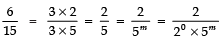=   which is of the form 2m· 5n.
∴  6/15 will have a terminating decimal expansion.

(ix) 35/50
∵ 50 = 2 × 5 × 5 = 21 × 52, which is of the form 2n · 5m.
∴ 35/50 will have a terminating decimal expansion.

(x) 77/210
∵  210 =  2 × 3 × 5 × 7 = 21 · 31 · 51 · 71,
which is not of the form of 2n · 5m.
∴  77/ 210 will have a non-terminating repeating decimal expansion.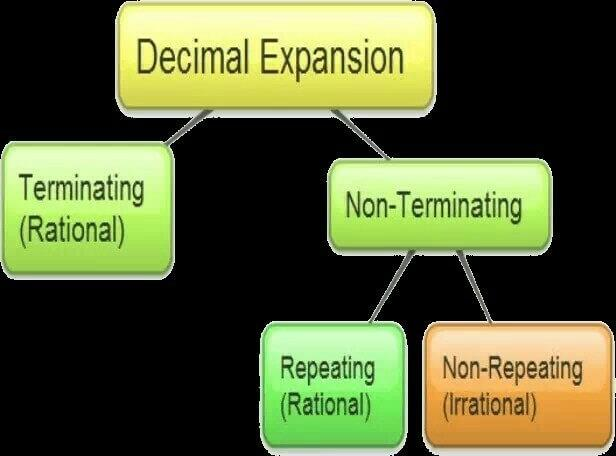Fig: Decimal expression of rational numbers

Ques 2: Write down the decimal expansions of those rational numbers in Question 1 above which have terminating decimal expansions.
(i)  13/ 3125
(ii) 17/18
(iii) 64/455
(iv) 15/1600
(v) 29/343
(vi)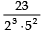(vii)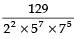(viii) 6/15
(ix) 35/50
(x) 77/ 210
Sol:
(i) 13/3125
We have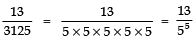Multiplying and dividing both sides, by 25, we have: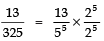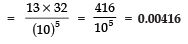(ii) 17/18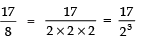Multiplying and dividing by 53, we have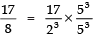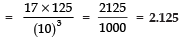(iii) 64/455 represents non-terminating repeating decimal expansion.

(iv) 15/1600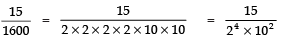Multiplying and dividing by 54, we have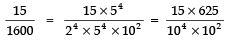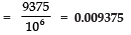(v) 29/343 , represents a non-terminating repeating decimal expansion.

(vi)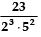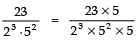=   |Multiplying and dividing by 5. =  = 0.115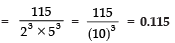(vii)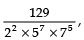represents a non-terminating repeating decimal expansion.

(viii) 6/15
6/15 =  2/5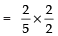|Multiplying and dividing by 2,

= 4/10   = 0.4

(ix) 35/50
35/50 = 0.7

(x) 77/ 210 , represents a non-terminating repeating decimal expansion.

Ques 3: The following real numbers have decimal expansions as given below. In each case, decide whether they are rational or not. If they are rational, and of the form, what can you say about the prime factors of q?
(i) 43.123456789
(ii) 0.120120012000120000...
(iii)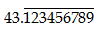Sol:
(i) 43.123456789
∵ The given decimal expansion terminates.
∴ It is a rational of the form p/q
⇒ p/q = 43.123456789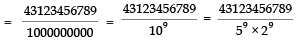Here, p  = 43.123456789  and
q = 29 × 59
∴ Prime factors of q are 29 and 59.

(ii) 0.120120012000120000 .....
∵ The given decimal expansion is neither terminating nor non-terminating repeating,
∴ It is not a rational number.

(iii)∵ The given decimal expansion is non-terminating repeating.
∴ It is a rational number.
Let p/q = x = 43.123456789 ......(1)
Multiplying both sides by 1000000000, we have
1000000000 x = 43.123456789  ...(2)
Subtracting (1) from (2), we have:
(1000000000 x) – x = (43.123456789) – 43.123456789
⇒ 999999999 x  = 43.123456746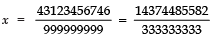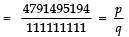Here, p =  4791495194
and  q  = 111111111, which is not of form 2n· 5m
i.e., the prime factors of q are not of form 2n· 5m.

Offer running on EduRev: Apply code STAYHOME200 to get INR 200 off on our premium plan EduRev Infinity!

132 docs

,

,

,

,

,

,

,

,

,

,

,

,

,

,

,

,

,

,

,

,

,

;